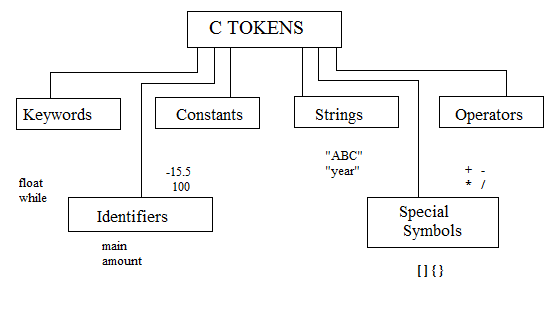# Binary Operators :

Binary operators are the most basic and common operators. These operators require two operands and an operator between them.

The following table shows the binary operators and their meanings :

Binary Operator Meaning
- Subtraction of two numbers
* Multiplication of two numbers
/ Division of a number by another
% Modulo of a number by another

In division the operands can be of integer or floating point type. If any one of the operands is floating point type, the result will be of floating-point type. If both the operands are integers the result will be integer.

However, the modulo operation cannot be done with floating point type. Both the operands for the modulo operator must be integer type. It returns the remainder as an integer type. The result of modulo division will take the sign of the first operand.

The multiplicative expressions that include the multiplication, division and modulo operators have the highest priority among binary operators. The additive expressions that include the addition and subtraction operators have lower priority than multiplicative expressions. Therefore, they are evaluated after the multiplicative expressions.

# Strings in C Language :

A String is a sequence of characters that is treated as a data item.

The group of characters, digits, and symbols enclosed within quotation marks is called as "string". The sting is always declared as a character array. To manipulate text such as words and sentences normally strings are used. Every string is terminated with '\0' (NULL) character. The NULL character is a byte with all bits at logic zero. Hence, its decimal value is zero.

` Example : char name[] = {'I', 'N', 'D', 'I', 'A', '\0' }; `
Character Strings are often used to build meaningful and readable programs.

The common operations performed on character strings include :